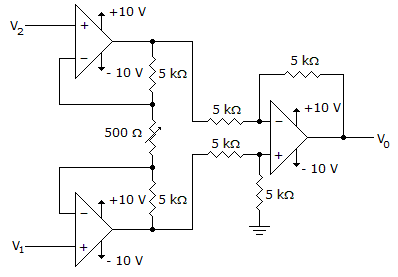# Electronic Devices - Op-Amp Applications - Discussion

Discussion Forum : Op-Amp Applications - General Questions (Q.No. 1)
1.
Calculate the output voltage for this circuit when V1 = 2.5 V and V2 = 2.25 V.–5.25 V
2.5 V
2.25 V
5.25 V
Explanation:
No answer description is available. Let's discuss.
Discussion:
22 comments Page 1 of 3.

Suba said:   7 months ago
Vo = (1+2R/Rp)v1-v2.

Raghuraj Gautam said:   2 years ago
Thanks for explaining.
(1)

Charan said:   5 years ago
Why they took 2R1 in the formula?

Anyone explain me.
(1)

Harsh tyagi said:   6 years ago
First apply the nodal equation and find the output of first two op-amp. The upper one has output -.25 volt. and the lower one has 5 volt.

Sanjay said:   7 years ago
Asking a normal q here. When amplifier at v1 and v2 have supply voltage +10 &-10v then how v1 and v2 amplified out can use on the third opamp?

Sachin said:   7 years ago
v0 =A * (V2 - V1),

A = 1 + (2 * 5k)/500.

Prachi Tewari said:   7 years ago
@Arnesh Sen answer is correct .

Husain Kapadia said:   8 years ago
For those, who don't want to remember the formula.

Consider the above op-amp to be 2 having o/p Vo2 and below op-amp to be 1 having o/p Vo1.

Since the op-amps are ideal we assume no currents flow into the input terminals, so drop across the 500 Ohm resistor is (V1-V2).

Current flowing through the 500 Ohm resistor is 0.5 mA.

Vo1 = V1 + drop across 5k Ohm = 5V (simple KVL path).
Vo2 = - (drop across 5k Ohm - V2) = -0.25 V (simple KVL path).

These o/ps Vo1 and Vo2 act as i/p to the third op-amp.

Apply superposition,

Vo = Vo1' + Vo2'

Consider Vo2 to be GND.

Vo1' = (1 + Rf/R1) * Vo1 * (5/10) = 5V
Vo2' = -Rf/R1*Vo2 = 0.25V

Vo = 5.25V
(8)

Wasim said:   8 years ago
Is it instrumentation amplifier?

Akshay said:   8 years ago
@Arnesh.

How is the three resistances are connected in series?
(1)